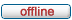# forum.alglib.net

ALGLIB forum
 It is currently Sat Jul 02, 2022 11:06 am

 All times are UTC

### Forum rules

1. This forum can be used for discussion of both ALGLIB-related and general numerical analysis questions
2. This forum is English-only - postings in other languages will be removed.

 Print view Previous topic | Next topic
Author Message
 Post subject: Does ALGLib work with Delphi 10.3 for Linux?Posted: Mon Jan 20, 2020 8:14 amJoined: Mon Jan 20, 2020 8:09 am
Posts: 2
I am able to compile when I change the following in the XALGLIB.pas wrapoer

Code:
{\$IFDEF FPC}
uses {\$IFDEF MSWINDOWS}Windows{\$ELSE}Dynlibs{\$ENDIF}, Math, StrUtils;
{\$ELSE}
//uses {\$IFDEF MSWINDOWS}Windows{\$ELSE}SysUtils{\$ENDIF}, Math, StrUtils, AnsiStrings;
uses Math, StrUtils, AnsiStrings;
{\$ENDIF}

Delphi is able to compile under Linux 64, but I get runtime error when I try to run the binary on my Linux box running 19.04 Ubuntu.

Thanks!

TopPost subject: Re: Does ALGLib work with Delphi 10.3 for Linux?Posted: Tue Jan 21, 2020 2:01 pmSite Admin

Joined: Fri May 07, 2010 7:06 am
Posts: 873
Hi!

A few questions:

1) did you put ALGLIB binary into same directory as your app binary file?
2) when exactly runtime error happens - in the xalglib.pas initialization section? or when you call some ALGLIB function?
3) what exactly kind of error you get - some standard Delphi exception? ALGLIB usually puts short textual description into Exception.Message - is it present?

Sergey

TopPost subject: Re: Does ALGLib work with Delphi 10.3 for Linux?Posted: Tue Jan 28, 2020 2:38 amJoined: Mon Jan 20, 2020 8:09 am
Posts: 2
Hi

1. Yes I have copied alglib316_64hpc.so to the directory.

2. Error inside ALGLib function Str2Vector

Code is the example code in ALGLib. I have added WriteLn to find out where the error occurs.
Code:
program Project3;

{\$APPTYPE CONSOLE}

{\$R *.res}

uses
System.SysUtils,
Classes,
Math,
xalglib;

var
a: Tsparsematrix;
b: TVector;
x0: TVector;
s: TVector;
x: TVector;
state: Tminqpstate;
rep: Tminqpreport;

begin
try
Writeln('Hello World');
a:=nil;
state:=nil;

//
// This example demonstrates minimization of F(x0,x1) = x0^2 + x1^2 -6*x0 - 4*x1,
// with quadratic term given by sparse matrix structure.
//
// Exact solution is [x0,x1] = [3,2]
//
// We provide algorithm with starting point, although in this case
// (dense matrix, no constraints) it can work without such information.
//
// IMPORTANT: this solver minimizes  following  function:
//     f(x) = 0.5*x'*A*x + b'*x.
// Note that quadratic term has 0.5 before it. So if you want to minimize
// quadratic function, you should rewrite it in such way that quadratic term
// is multiplied by 0.5 too.
//
// For example, our function is f(x)=x0^2+x1^2+..., but we rewrite it as
//     f(x) = 0.5*(2*x0^2+2*x1^2) + ....
// and pass diag(2,2) as quadratic term - NOT diag(1,1)!
//
Writeln('1');
b:=Str2Vector('[-6,-4]');  // <--- runtime error here
Writeln('1.1');
x0:=Str2Vector('[0,1]');
Writeln('1.2');
s:=Str2Vector('[1,1]');
Writeln('1.3');

Writeln('2');
// initialize sparsematrix structure
sparsecreate(2, 2, 0, a);
sparseset(a, 0, 0, 2.0);
sparseset(a, 1, 1, 2.0);

// create solver, set quadratic/linear terms
Writeln('3');
minqpcreate(2, state);
minqpsetlinearterm(state, b);
minqpsetstartingpoint(state, x0);

// Set scale of the parameters.
// It is strongly recommended that you set scale of your variables.
// Knowing their scales is essential for evaluation of stopping criteria
// and for preconditioning of the algorithm steps.
//
// NOTE: for convex problems you may try using minqpsetscaleautodiag()
//       which automatically determines variable scales.
Writeln('4');
minqpsetscale(state, s);

//
// Solve problem with BLEIC-based QP solver.
//
// This solver is intended for problems with moderate (up to 50) number
// of general linear constraints and unlimited number of box constraints.
// It also supports sparse problems.
//
// Default stopping criteria are used.
//
Writeln('5');
minqpsetalgobleic(state, 0.0, 0.0, 0.0, 0);
minqpoptimize(state);
minqpresults(state, x, rep);
WriteLn(Format('%s', [FormatVector(x,2)])); // EXPECTED: [3,2]

Writeln('6');
FreeAndNil(a);
Writeln('7');
FreeAndNil(state);
Writeln('8');

ExitCode:=0;

except
on E: Exception do
Writeln(E.ClassName, ': ', E.Message);
end;

end.

Note that I also had to comment out and change the following in XALGLib.pas itself, otherwise I would get the System.Sysutils redeclared error.

Code:
{\$IFDEF FPC}
uses {\$IFDEF MSWINDOWS}Windows{\$ELSE}Dynlibs{\$ENDIF}, Math, StrUtils;
{\$ELSE}
//uses {\$IFDEF MSWINDOWS}Windows{\$ELSE}SysUtils{\$ENDIF}, Math, StrUtils, AnsiStrings;
uses Math, StrUtils, AnsiStrings;
{\$ENDIF}

3. Output
Code:
lliew@beowulf:~/PAServer/scratch-dir/laure-Beowulf/Project3\$ ./Project3
Hello World
1
free(): invalid pointer
Aborted (core dumped)
lliew@beowulf:~/PAServer/scratch-dir/laure-Beowulf/Project3\$ ls
Project3  alglib316_64hpc.so

TopDisplay posts from previous: All posts1 day7 days2 weeks1 month3 months6 months1 year Sort by AuthorPost timeSubject AscendingDescending

 All times are UTC

#### Who is online

Users browsing this forum: Google [Bot] and 2 guests

 You cannot post new topics in this forumYou cannot reply to topics in this forumYou cannot edit your posts in this forumYou cannot delete your posts in this forumYou cannot post attachments in this forum

Search for: